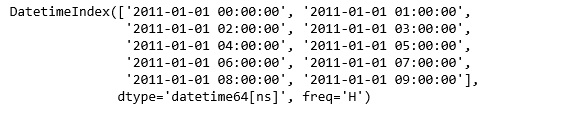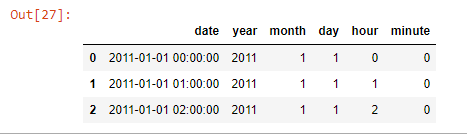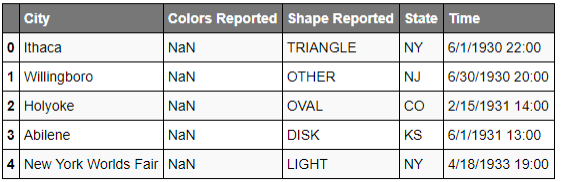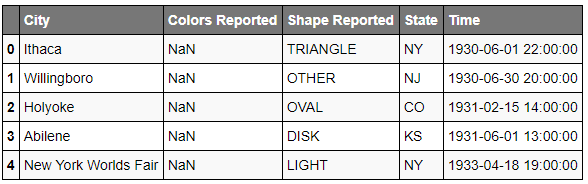# Python | Working with date and time using Pandas

• Difficulty Level : Medium
• Last Updated : 10 Mar, 2022

While working with data, encountering time series data is very usual. Pandas is a very useful tool while working with time series data.

Pandas provide a different set of tools using which we can perform all the necessary tasks on date-time data. Let’s try to understand with the examples discussed below.

Code #1: Create a dates dataframe

## Python3

 `import` `pandas as pd`   `# Create dates dataframe with frequency  ` `data ``=` `pd.date_range(``'1/1/2011'``, periods ``=` `10``, freq ``=``'H'``)`   `data`

Output:Code #2: Create range of dates and show basic features

## Python3

 `# Create date and time with dataframe` `data ``=` `pd.date_range(``'1/1/2011'``, periods ``=` `10``, freq ``=``'H'``)`   `x ``=` `pd.datetime.now()` `x.month, x.year`

Output:

`(9, 2018)`

Datetime features can be divided into two categories. The first one time moments in a period and second the time passed since a particular period. These features can be very useful to understand the patterns in the data.

Divide a given date into features –

pandas.Series.dt.year returns the year of the date time.
pandas.Series.dt.month returns the month of the date time.
pandas.Series.dt.day returns the day of the date time.
pandas.Series.dt.hour returns the hour of the date time.
pandas.Series.dt.minute returns the minute of the date time.
Refer all datetime properties from here.

Code #3: Break date and time into separate features

## Python3

 `# Create date and time with dataframe` `rng ``=` `pd.DataFrame()` `rng[``'date'``] ``=` `pd.date_range(``'1/1/2011'``, periods ``=` `72``, freq ``=``'H'``)`   `# Print the dates in dd-mm-yy format` `rng[:``5``]`   `# Create features for year, month, day, hour, and minute` `rng[``'year'``] ``=` `rng[``'date'``].dt.year` `rng[``'month'``] ``=` `rng[``'date'``].dt.month` `rng[``'day'``] ``=` `rng[``'date'``].dt.day` `rng[``'hour'``] ``=` `rng[``'date'``].dt.hour` `rng[``'minute'``] ``=` `rng[``'date'``].dt.minute`   `# Print the dates divided into features` `rng.head(``3``)`

Output:Code #4: To get the present time, use Timestamp.now() and then convert timestamp to datetime and directly access year, month or day.

## Python3

 `# Input present datetime using Timestamp` `t ``=` `pandas.tslib.Timestamp.now()` `t`

`Timestamp('2018-09-18 17:18:49.101496')`

## Python3

 `# Convert timestamp to datetime` `t.to_datetime()`

`datetime.datetime(2018, 9, 18, 17, 18, 49, 101496)`

## Python3

 `# Directly access and print the features` `t.year` `t.month` `t.day` `t.hour` `t.minute` `t.second`

```2018
8
25
15
53```

Let’s analyze this problem on a real dataset uforeports.

## Python3

 `import` `pandas as pd`   `url ``=` `'http://bit.ly/uforeports'`   `# read csv file` `df ``=` `pd.read_csv(url)           ` `df.head()`

Output:## Python3

 `# Convert the Time column to datetime format` `df[``'Time'``] ``=` `pd.to_datetime(df.Time)`   `df.head()`## Python3

 `# shows the type of each column data` `df.dtypes`

```City                       object
Colors Reported            object
Shape Reported             object
State                      object
Time               datetime64[ns]
dtype: object```

## Python3

 `# Get hour detail from time data` `df.Time.dt.hour.head()`

```0    22
1    20
2    14
3    13
4    19
Name: Time, dtype: int64```

## Python3

 `# Get name of each date` `df.Time.dt.weekday_name.head()`

```0     Sunday
1     Monday
2     Sunday
3     Monday
4    Tuesday
Name: Time, dtype: object```

## Python3

 `# Get ordinal day of the year` `df.Time.dt.dayofyear.head()`

```0    152
1    181
2     46
3    152
4    108
Name: Time, dtype: int64```

My Personal Notes arrow_drop_up
Recommended Articles
Page :